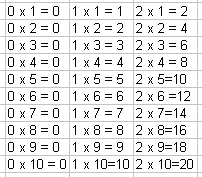Name: ___________________Date:___________________

 Email us to get an instant 20% discount on highly effective K-12 Math & English kwizNET Programs!

### Grade 4 - Mathematics3.2 Multiplication Facts 0-5Directions: Review the time table and answer the following questions. Also write ten examples of your own and multiplication facts for 0,1,2,3,4,5 on a sheet of paper.Name: ___________________Date:___________________

### Grade 4 - Mathematics3.2 Multiplication Facts 0-5

 Q 1: 0 x 5 =5100 Q 2: 2 x 8 =16410 Q 3: 3 x 3 =609 Q 4: 4 x 9=281336 Q 5: 5 x 3 =1582 Q 6: 6 x 8 =494842 Q 7: 7 x 2 =181114 Q 8: 8 x 3 =212724 Question 9: This question is available to subscribers only! Question 10: This question is available to subscribers only!